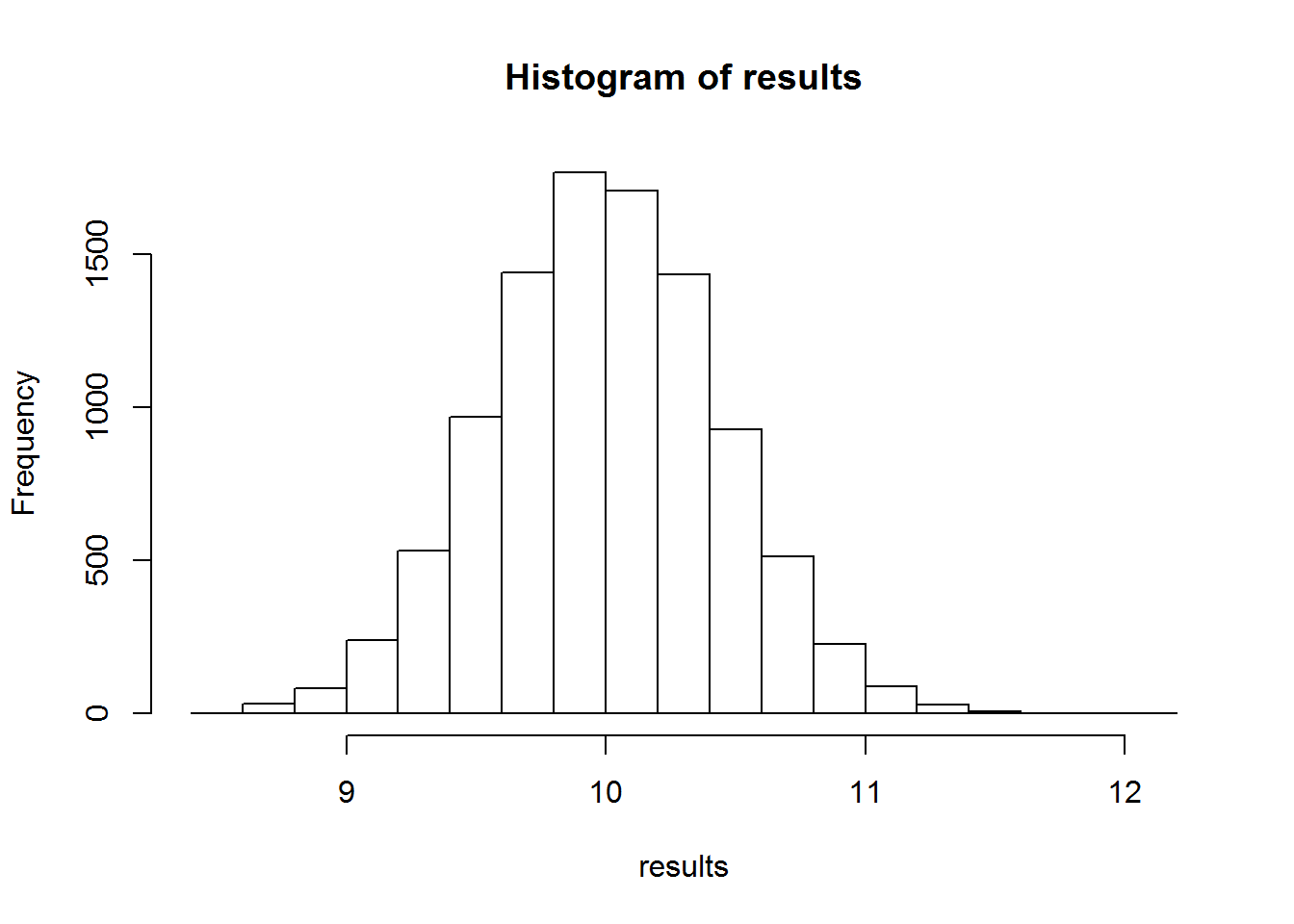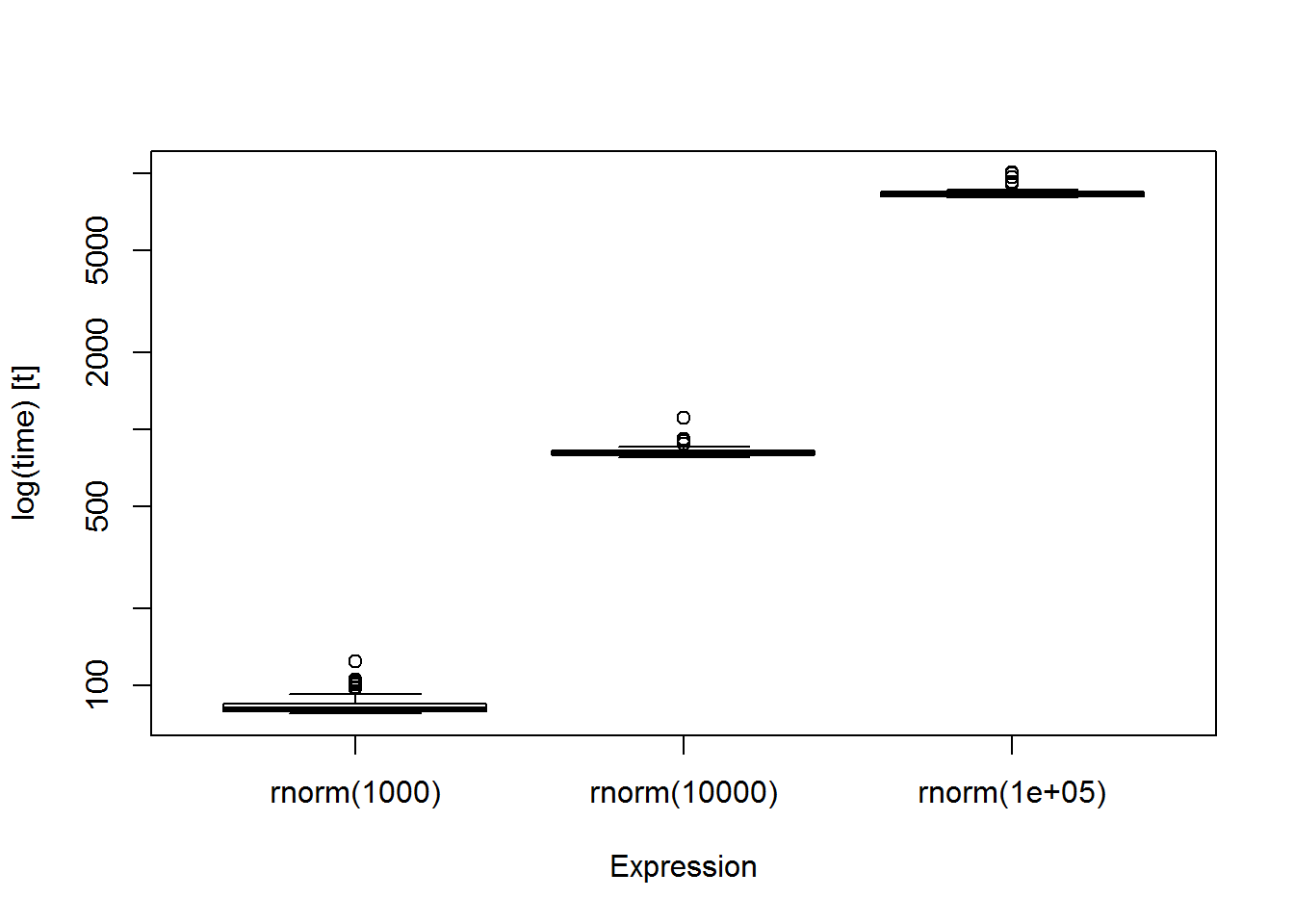## Getting Started

Install and load the following packages:

• gapminder
• dplyr
• microbenchmark

The for loop is used in the following form:

for(counter) {#action steps}

### Loops Two Ways

1. Loop over indices e.g.:
for(i in 1:10){
print(i)
}
1. Loop over elements of a data structure e.g.:
for(i in head(gapminder$country)) { print(i) } • Related: Loop over names/levels of a factor for(i in levels(gapminder$country)){
print(i)
}

## Why Loop?

### 1. Resampling Statistics or Simulation (e.g. permutation tests, bootstrapping)

In the following example, we will simulate the central limit theorem by randomly sampling and taking the mean (over 10000 iterations) from a poisson distribution.

results <- rep(NA, 10000)
for(i in 1:10000){
a <- rpois(50, lambda=10)
results[i] <-mean(a)
}
hist(results)Bootstrapping: Resampling with replacement to determine confidence intervals on a statistic, e.g. the mean. Let’s get CIs on the mean of the gdpPercap factor of gapminder. The basic steps involve:

1. Sampling with replacement.
2. Calculating the statistic you wish from this sample you’ve obtained.
3. Repeat over x iterations (generally >1000)
4. Estimate sample distribution to determine confidence intervals for statistic of interest.
gdpresults <- rep(NA, 10000)
for(i in 1:10000){
resample <- sample(gapminder$gdpPercap, replace=T) gdpresults[i] <- mean(resample) } quantile(gdpresults, c(0.025, 0.975)) # get 95% CI ## 2.5% 97.5% ## 6758.579 7687.294 ### 2. Processing/Analyzing/Visualizing large and/or distributed datasets You may want to perform the same analysis on different subsets/columns of a dataframe/list. This is particularly useful if the analysis has multiple components. #loop over subsets countries <- levels(gapminder$country)
results <- rep(NA, length(countries))
for(i in 1:length(countries)){
mod <- lm(lifeExp ~ gdpPercap, subset(gapminder, country==countries[i]))
results[i]<-summary(mod)$r.squared } #loop over certain columns (lm on column ~ year) responses <- names(gapminder)[4:6] results <- rep(NA, length(responses)) for(i in 1:length(responses)){ myformula <- paste(responses[i],'~year') mod <- lm(as.formula(myformula), data=gapminder) results[i]<-summary(mod)$r.squared
}
results[1:3]
##  0.18975714 0.00677462 0.05167350

We can make and save lots of separate plots. First, create an empty folder and set it as your working directory. The loop below will generate many plots in that folder.

for(i in 1:length(countries)){
mypath <- file.path(getwd(), paste(countries[i], '_', paste('00',i,sep=''), ".jpg", sep = ""))
jpeg(file=mypath, width=4, height=3.5, units='in', res=150)
par(las=1, bty='n', family='Times', tck=0.02)
plot(gdpPercap~year,subset(gapminder, country==countries[i]), xlab='year', ylab='gdp per capita (units)', type='l', ylim=c(1,10000))
dev.off()
} 

While the concept of for looping is very powerful, it is often not the best choice available.

Here you are attempting to create a new column with rounded values of a column using a for loop. (Don’t do this!)

for(i in 1:length(gapminder$pop)){ gapminder$popround[i] <- round(gapminder$pop[i], -3) } head(gapminder) ## Source: local data frame [6 x 7] ## ## country continent year lifeExp pop gdpPercap popround ## (fctr) (fctr) (int) (dbl) (int) (dbl) (dbl) ## 1 Afghanistan Asia 1952 28.801 8425333 779.4453 8425000 ## 2 Afghanistan Asia 1957 30.332 9240934 820.8530 9241000 ## 3 Afghanistan Asia 1962 31.997 10267083 853.1007 10267000 ## 4 Afghanistan Asia 1967 34.020 11537966 836.1971 11538000 ## 5 Afghanistan Asia 1972 36.088 13079460 739.9811 13079000 ## 6 Afghanistan Asia 1977 38.438 14880372 786.1134 14880000 Do this instead (vectorized!) gapminder$popround <- round(gapminder$pop, -3) Recoding Values Here we want to change all values of iris’ Group Value to 1 if Sepal Length is between 5 and 6, and set to 2 if greater than or equal to 6 (and set to 0 otherwise). Don’t do this: iris$sep.group <- 0
for (i in 1:length(iris$Sepal.Length)){ if(iris$Sepal.Length[i] > 5 & iris$Sepal.Length[i] < 6){ iris$sep.group[i] <- 1
}
if(iris$Sepal.Length[i] >= 6){ iris$sep.group[i] <- 2
}
}
head(iris)
##   Sepal.Length Sepal.Width Petal.Length Petal.Width Species sep.group
## 1          5.1         3.5          1.4         0.2  setosa         1
## 2          4.9         3.0          1.4         0.2  setosa         0
## 3          4.7         3.2          1.3         0.2  setosa         0
## 4          4.6         3.1          1.5         0.2  setosa         0
## 5          5.0         3.6          1.4         0.2  setosa         0
## 6          5.4         3.9          1.7         0.4  setosa         1

It is more efficient to use ifelse(<condition>, <yes>, <no>) which can also be nested:

iris$sep.group <- ifelse(iris$Sepal.Length > 6, 2, ifelse(iris$Sepal.Length > 5, 1, 0)) head(iris) ## Sepal.Length Sepal.Width Petal.Length Petal.Width Species sep.group ## 1 5.1 3.5 1.4 0.2 setosa 1 ## 2 4.9 3.0 1.4 0.2 setosa 0 ## 3 4.7 3.2 1.3 0.2 setosa 0 ## 4 4.6 3.1 1.5 0.2 setosa 0 ## 5 5.0 3.6 1.4 0.2 setosa 0 ## 6 5.4 3.9 1.7 0.4 setosa 1 Do not use for loops to apply summary functions (mean, max, min etc.) to subsets of your data. countries <- levels(gapminder$country)
cmeans <- rep(NA, length(countries))
for(i in 1:length(countries)){
data <- subset(gapminder, country==countries[i])
cmeans[i] <- mean(data$pop) } head(cmeans) ##  15823715 2580249 19875406 7309390 28602240 14649313 dplyr has a summarise() function for this purpose by.country <- group_by(gapminder, country) summ.country <- summarise(by.country, meanpop = round(mean(pop)/1000,-3)) head(summ.country) ## Source: local data frame [6 x 2] ## ## country meanpop ## (fctr) (dbl) ## 1 Afghanistan 16000 ## 2 Albania 3000 ## 3 Algeria 20000 ## 4 Angola 7000 ## 5 Argentina 29000 ## 6 Australia 15000 #### The Apply Functions In base R, the apply family is very useful in place of writing your own loops: help("apply") apply(gapminder[,4:6], 2, 'mean') # applied to columns head(apply(iris[,1:4], 1, 'sum')) #applied to rows help(tapply) # good for dataframes or separate vectors which can be ragged (different sizes) tapply(gapminder$lifeExp, gapminder$country, FUN='mean') # tapply(vector, factor, function) tapply(gapminder$lifeExp, gapminder$country, FUN=function(x) round(mean(x),-1)) # can write your own functions to bue used with apply & co. can be done inside or outside the loop Note that you can write your own function to be used with the apply family, either inside or outside the loop. In this example, we will use tapply() to normalize all the values of gapminder$pop based on country. Then we will use lapply() which works on lists to apply our functions.

poppercent <- tapply(gapminder$pop, gapminder$country, function(x) x/max(x))
#this will give a list of lists, ordered by country
#now we can use lapply on this list
lapply(poppercent, mean) #to find the mean
lapply(poppercent, function(x) sd(x)/sqrt(length(x))) #to find the SEM

#### How to Time Your Code

1. Enclose in system.time()
system.time(mean(1:1000000))
##    user  system elapsed
##    0.02    0.00    0.01
1. For big chunks, determine the processing time of code with proc.time()
pmt <- proc.time()
mean(1:1000000)
##  500000.5
proc.time() - pmt
##    user  system elapsed
##       0       0       0
1. Using microbenchmark, it will be a more accurate estimate as it samples the action many times (default = 100, but can change as desired). This will take longer but can be useful if choosing between two methods that you will use extensively.
microbenchmark(mean(1:1000000))
## Unit: milliseconds
##           expr      min     lq     mean   median       uq      max neval
##  mean(1:1e+06) 2.144687 2.5918 11.58111 3.735134 4.797427 47.87291   100

You can also pass it multiple things to compare speeds:

rnormtime <- microbenchmark(rnorm(1000), rnorm(10000), rnorm(100000), times=50)
boxplot(rnormtime)Here are some basic principles to follow when looping:

1.Initialize your data structures rather than growing them as you loop (avoid appending to a given structure; pre-allocate space instead and then access!)

Let’s look at an example here of growing vs. pre-allocating/accessing:

hit <- NA
system.time (for(i in 1:100000){if(runif(1) < 0.3) hit[i] <- T})
##    user  system elapsed
##    1.97    0.04    2.00
hit2 <- rep(NA, 100000)
system.time (for(i in 1:100000){if(runif(1) < 0.3) hit2[i] <- T})
##    user  system elapsed
##    0.22    0.00    0.22

2.Take advantage of vectorized operations when possible and take the work outside of loops.

Note, sometimes more code is not always a bad thing, especially if your primary goal is time saving in the computations, especially as you begin to work with increasingly large datasets.

Here we will try to denote all years in which lifeExp of a country decreased as a “bad” year.

First with a for loop…

pmt <- proc.time()
gapminder$badyear <- NA rows <- length(gapminder[,1]) for(i in 2:rows){ if((gapminder$lifeExp[i] < gapminder$lifeExp[i-1]) & (gapminder$country[i]==gapminder$country[i-1])){ gapminder$badyear[i] <- 'bad'
}
}
proc.time() - pmt
summary(factor(gapminder$badyear)) And without: start <- proc.time() gapminder$lifeExpnext <- NA
rows <- length(gapminder[,1])
# create a column where, for each year, the next year's lifeExp is indicated
gapminder$lifeExpnext[1:rows-1] <- gapminder$lifeExp[2:rows]
gapminder$countrynext <- NA # in order to keep track of country (don't want to compare two different countries) gapminder$countrynext[1:rows-1] <- as.character(gapminder$country[2:rows]) # the vectorized component gapminder$badyear <- ifelse(gapminder$countrynext==gapminder$country & gapminder$lifeExpnext < gapminder$lifeExp, "bad", NA)
proc.time() - start
##    user  system elapsed
##       0       0       0

3.Indexing vectors is faster than indexing dataframes.

#in a dataframe
results <- data.frame(t1=rep(NA,1000), pval=NA)

microbenchmark(
for(i in 1:1000){
a <- rnorm(50, 10)
grp <- rep(1:2, each = length(a)/2)
stat <- t.test(a~grp)
results$t1[i] <- stat$statistic
results$pval[i] <- stat$p.value
}, times = 1
)
## Unit: milliseconds
##                                                                                                                                                                                      expr
##  for (i in 1:1000) {     a <- rnorm(50, 10)     grp <- rep(1:2, each = length(a)/2)     stat <- t.test(a ~ grp)     results$t1[i] <- stat$statistic     results$pval[i] <- stat$p.value }
##       min       lq     mean   median       uq      max neval
##  931.6818 931.6818 931.6818 931.6818 931.6818 931.6818     1
#as two vectors
t1 <- rep(NA, 1000)
pval <- rep(NA, 1000)

microbenchmark(
for(i in 1:1000){
a <- rnorm(50, 10)
grp <- rep(1:2, each = length(a)/2)
stat <- t.test(a ~ grp)
t1[i] <- stat$statistic pval[i] <- stat$p.value
}, times = 1
)
## Unit: milliseconds
##                                                                                                                                                                      expr
##  for (i in 1:1000) {     a <- rnorm(50, 10)     grp <- rep(1:2, each = length(a)/2)     stat <- t.test(a ~ grp)     t1[i] <- stat$statistic pval[i] <- stat$p.value }
##       min       lq     mean   median       uq      max neval
##  903.2607 903.2607 903.2607 903.2607 903.2607 903.2607     1
#Small difference, but can save time on larger datasets

### Resources (free online!)

Patrick Burns’ R Inferno: http://www.burns-stat.com/pages/Tutor/R_inferno.pdf